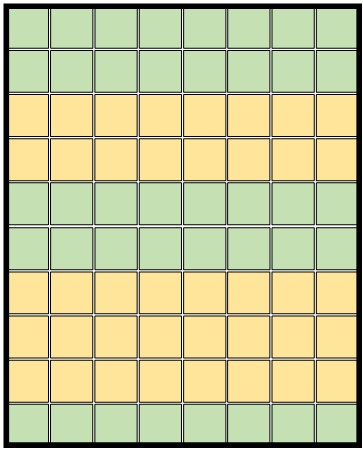# How to implement an irregular mean-pooling？

Suppose I have 2-D tensor as shown below:I wanna reserve the green blocks and compute mean vector over each yellow block with indexes (e.g., [[2,3], [6,7,8]])

How to implement these operations with pytorch? pls help me

I’m unsure if there is a cleaner and better approach, but you might use `tensor.scatter_reduce_` with the `reduce="mean"` argument and slice the output. This would waste compute since you would be moving the unwanted values to the end of the result, but might be faster than iterating the indices.
This code should work:

``````# setup
x = torch.randn(10, 8)

# create indices
idx = [[2,3], [6,7,8]]
indices = torch.full((x.size(0),), len(idx))
for i, l in enumerate(idx):
indices[l] = i
print(indices)
# tensor([2, 2, 0, 0, 2, 2, 1, 1, 1, 2])

indices = indices.unsqueeze(1).expand(-1, 8)
out = torch.zeros(3, 8).scatter_reduce_(0, indices, x, reduce="mean", include_self=False).mean(dim=1)[:2]

reference = []
for i in idx:
reference.append(x[i, :].mean())
reference = torch.tensor(reference)

print((reference - out).abs().max())
# tensor(1.4901e-08)
``````
1 Like

Thx, it seems cool !